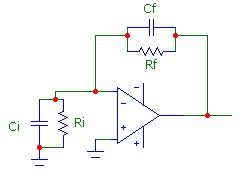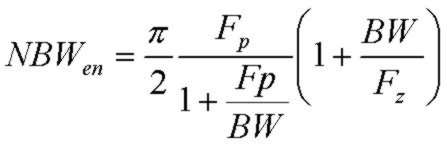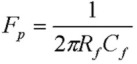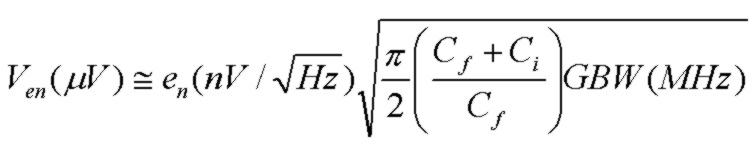# Formulae for Transimpedance Amplifier Noise

July 02, 2012This summary page provides formulae for the exact and approximate output voltage noise for the transimpedance circuit shown to the right. The noise is the total noise, integrated over all frequency. The approximations are discussed here with more detailed background information available here.

An exact noise calculator also provides cumulative noise (up to any frequency f) results.

## Exact Results:

The exact expression for the total output voltage noise, summed over all frequencies, for the circuit shown above is:where en and in are the op-amp spectral voltage and current noise densities, N0 is the DC noise gain, Kb is Boltzmann's constant, T is the absolute temperature and Rf is the feedback resistance. NBWen is the noise bandwidth for the voltage noise contribution, and NBWth_in is the noise bandwidth for the current noise and resistors thermal noise contributions:The zero and pole frequencies of the noise gain and the basic "bandwidth" are:These expressions are exact and valid for any location of the zero and pole frequencies and for any op-amp GBW product, assuming a single-pole rolloff model for the op-amp open loop gain..

## Approximate Results: (valid for Fp<GBW, Fz<<GBW )

The total output voltage noise is the square-root of the squared sum (RMS) of each contributing uncorrelated noise voltage source.
Ven due to the op-amp voltage noise, Vin due to the op-amp current noise and Vth the thermal noise due to the combination of Rf and Ri:The thermal noise term is for T=293K (20C).
Notice that in this approximation, the op-amp voltage noise contribution Ven does not depend explicitly on Ri and Rf. In many cases, Ci>>Cf. Therefore reducing the input capacitance will lower the noise contribution from en.

Example: GBW=65MHz    en=7nV/√Hz    in=5fA/√Hz    Rf=50kΩ    Cf=1.35pF    Ri=200kΩ    Ci=12pF

Approx: Ven=222.4 µV    Vin=0.48 µV    Vth=61.2 µV    Vnout=230.7 µV

Exact:   Ven=218.2 µV    Vin=0.47 µV    Vth=59.9 µV    Vnout=226.2 µV

Results are within 2%.